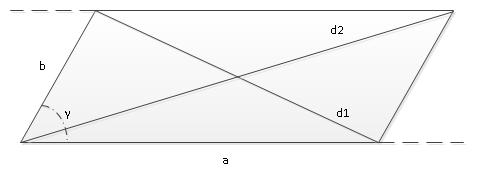# The diagonals of a parallelogram

Calculator computes the diagonals of a parallelogram and adjancent angles from side lengths and angle.This calculator computes the diagonals of a parallelogram and adjacent angles from side lengths and angles between sides.

It is done with the help of law of cosines

Check the picture. First, we use the law of cosines to find out d1, then we find the second angle of a parallelogram, which is , then we again use the law of cosines to find out d2. The remaining angles are found from the same law of cosines. After that, we find diagonal intersection angles using the fact that the sum of triangle angles is 180.#### The polygon diagonals of a parallelogram

°
Digits after the decimal point: 2
Diagonal d1

Diagonal d2

Second parallelogram angle

Angle between d1 and d2

Angle between a and d1

Angle between b and d1

Angle between a and d2

Angle between b and d2

URL copied to clipboard
PLANETCALC, The diagonals of a parallelogram